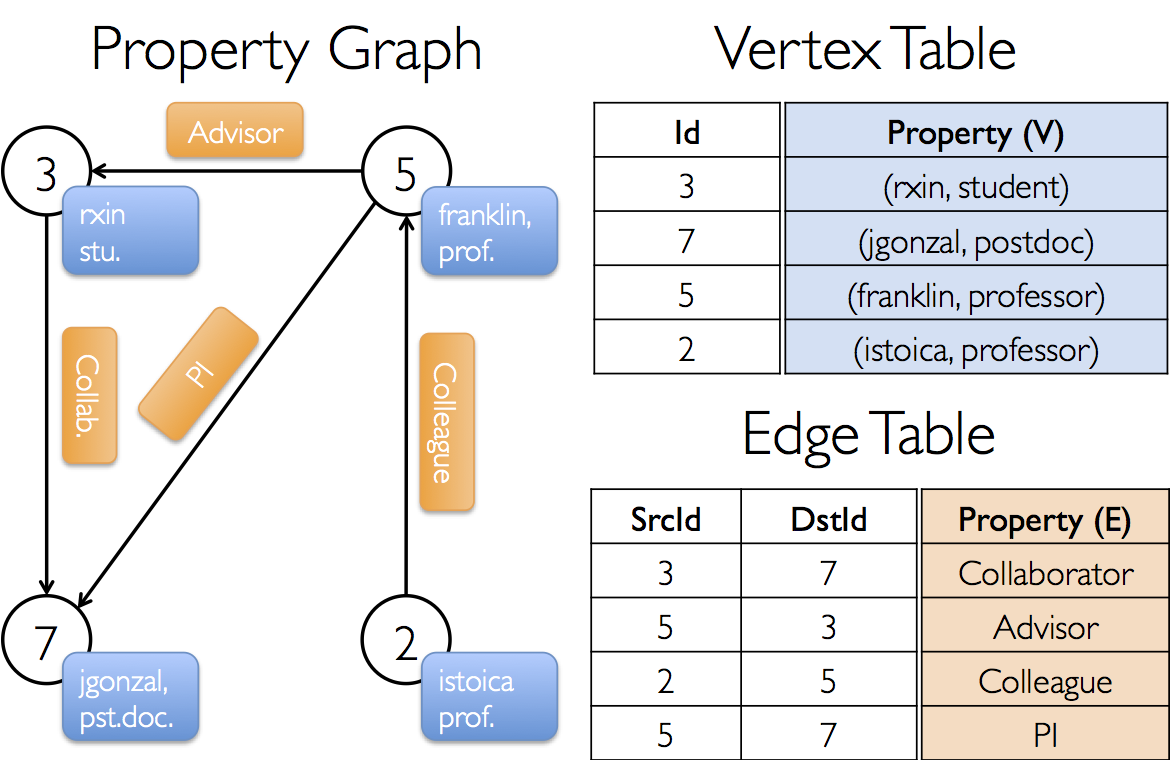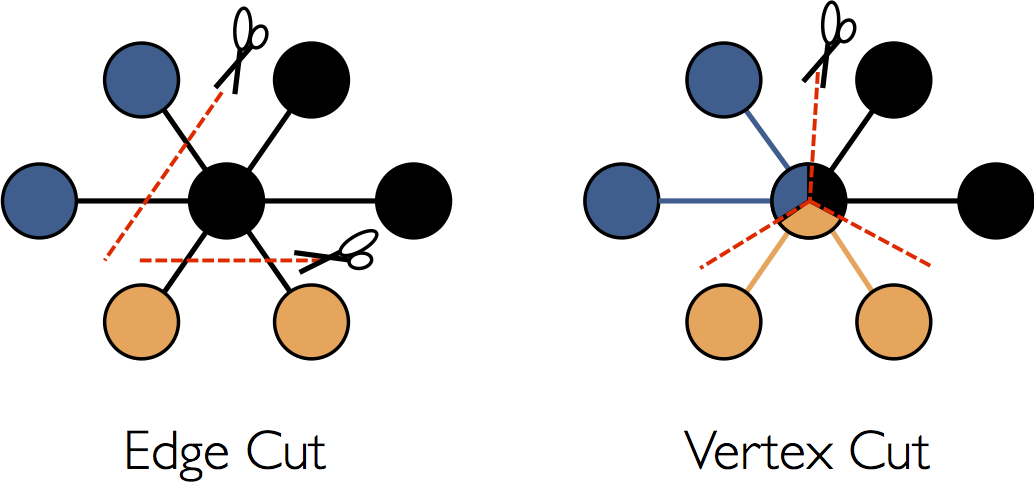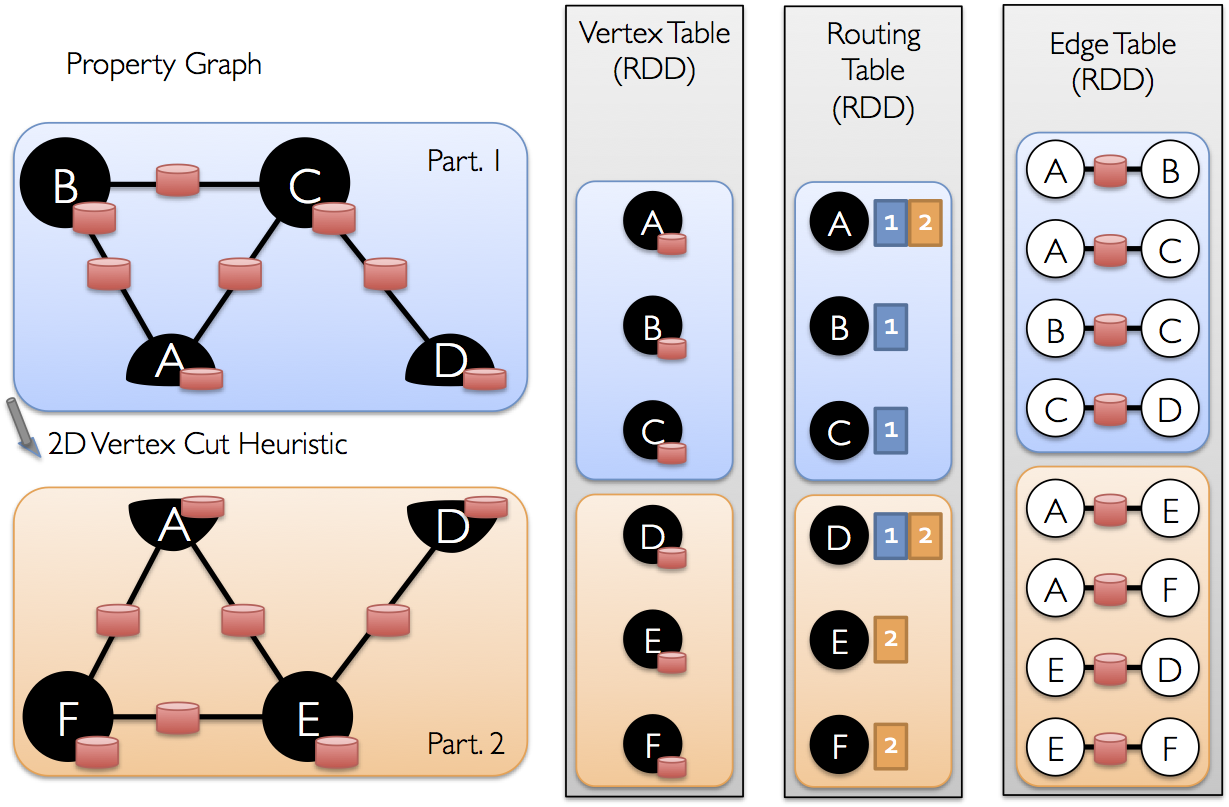2018-08-16

# 总览

GraphX 是 Spark 一个新的组件，专门用来表示图以及进行图的并行计算。 从一个高的角度来看，GraphX 通过重新定义了图的抽象概念来拓展了 RDD： 定向多图，其属性附加到每个顶点和边。

# 属性图 The Property Graph

``````class VertexProperty()
case class UserProperty(val name: String) extends VertexProperty
case class ProductProperty(val name: String, val price: Double) extends VertexProperty
// The graph might then have the type:
var graph: Graph[VertexProperty, String] = null
``````

``````class Graph[VD, ED] {
val vertices: VertexRDD[VD]
val edges: EdgeRDD[ED]
}
``````

VertexRDD[VD]EdgeRDD[ED] 类扩展并分别是 RDD[(VertexId, VD)]RDD[Edge [ED]] 的优化版本。 VertexRDD[VD] 和 EdgeRDD[ED] 都提供了围绕图形计算构建的附加功能，并利用了内部优化。 我们在顶点和边缘RDD部分中更详细地讨论了 VertexRDD 和 EdgeRDD API，但是现在它们可以被认为是 RDD[(VertexId，VD)]和 RDD[Edge[ED]] 形式的简单RDD。

# 属性图的例子``````val userGraph: Graph[(String, String), String]
``````

``````// 假设 SparkContext 已经被构建
val sc: SparkContext
// 为 vertices 创建一个 RDD
val users: RDD[(VertexId, (String, String))] =
sc.parallelize(Array((3L, ("rxin", "student")), (7L, ("jgonzal", "postdoc")),
(5L, ("franklin", "prof")), (2L, ("istoica", "prof"))))
// 为 edges 创建一个 RDD
val relationships: RDD[Edge[String]] =
sc.parallelize(Array(Edge(3L, 7L, "collab"),    Edge(5L, 3L, "advisor"),
Edge(2L, 5L, "colleague"), Edge(5L, 7L, "pi")))
// 定义一个默认的用户，来防止一个关系指向了不存在的用户
val defaultUser = ("John Doe", "Missing")
// 构建初步的 Graph
val graph = Graph(users, relationships, defaultUser)
``````

``````val graph: Graph[(String, String), String] // 上面构造的graph
// 计算所有是postdocs的用户数量
graph.vertices.filter { case (id, (name, pos)) => pos == "postdoc" }.count
// 计算所有 src > dst 的边的数量
graph.edges.filter(e => e.srcId > e.dstId).count
``````

``````graph.edges.filter { case Edge(src, dst, prop) => src > dst }.count
``````

``````SELECT src.id, dst.id, src.attr, e.attr, dst.attr
FROM edges AS e LEFT JOIN vertices AS src, vertices AS dst
ON e.srcId = src.Id AND e.dstId = dst.Id
``````

EdgeTriplet 继承了 Edges， 但是添加了 srcAttr 和 dstAttr 两个成员变量来分别表示源顶点和目标顶点的属性。 我们使用图的三元组视图可以得到一个能够呈现描述用户之间关系的字符串集合。

``````val graph: Graph[(String, String), String]
// Use the triplets view to create an RDD of facts.
val facts: RDD[String] =
graph.triplets.map(triplet =>
triplet.srcAttr._1 + " is the " + triplet.attr + " of " + triplet.dstAttr._1)
facts.collect.foreach(println(_))
``````

# 图运算符（Graph Operators）

``````val graph: Graph[(String, String), String]
// Use the implicit GraphOps.inDegrees operator
val inDegrees: VertexRDD[Int] = graph.inDegrees
``````

## 操作的摘要列表

``````/** Summary of the functionality in the property graph */
class Graph[VD, ED] {
// Graph 的信息 ===================================================================
val numEdges: Long
val numVertices: Long
val inDegrees: VertexRDD[Int]
val outDegrees: VertexRDD[Int]
val degrees: VertexRDD[Int]
// graph 的集合视角 =============================================================
val vertices: VertexRDD[VD]
val edges: EdgeRDD[ED]
val triplets: RDD[EdgeTriplet[VD, ED]]
// 缓存 graphs 的函数 ==================================================================
def persist(newLevel: StorageLevel = StorageLevel.MEMORY_ONLY): Graph[VD, ED]
def cache(): Graph[VD, ED]
def unpersistVertices(blocking: Boolean = true): Graph[VD, ED]
// 更改分区启发式  ============================================================
def partitionBy(partitionStrategy: PartitionStrategy): Graph[VD, ED]
// 变换顶点和边缘属性 ==========================================================
def mapVertices[VD2](map: (VertexId, VD) => VD2): Graph[VD2, ED]
def mapEdges[ED2](map: Edge[ED] => ED2): Graph[VD, ED2]
def mapEdges[ED2](map: (PartitionID, Iterator[Edge[ED]]) => Iterator[ED2]): Graph[VD, ED2]
def mapTriplets[ED2](map: EdgeTriplet[VD, ED] => ED2): Graph[VD, ED2]
def mapTriplets[ED2](map: (PartitionID, Iterator[EdgeTriplet[VD, ED]]) => Iterator[ED2]): Graph[VD, ED2]
// 修改 graph 结构 ====================================================================
def reverse: Graph[VD, ED]
def subgraph(
epred: EdgeTriplet[VD,ED] => Boolean = (x => true),
vpred: (VertexId, VD) => Boolean = ((v, d) => true))
: Graph[VD, ED]
def mask[VD2, ED2](other: Graph[VD2, ED2]): Graph[VD, ED]
def groupEdges(merge: (ED, ED) => ED): Graph[VD, ED]
// join RDDs 和 graph ======================================================================
def joinVertices[U](table: RDD[(VertexId, U)])(mapFunc: (VertexId, VD, U) => VD): Graph[VD, ED]
def outerJoinVertices[U, VD2](other: RDD[(VertexId, U)])
(mapFunc: (VertexId, VD, Option[U]) => VD2)
: Graph[VD2, ED]
// 有关相邻三元组的汇总信息 =================================================
def collectNeighborIds(edgeDirection: EdgeDirection): VertexRDD[Array[VertexId]]
def collectNeighbors(edgeDirection: EdgeDirection): VertexRDD[Array[(VertexId, VD)]]
def aggregateMessages[Msg: ClassTag](
sendMsg: EdgeContext[VD, ED, Msg] => Unit,
mergeMsg: (Msg, Msg) => Msg,
tripletFields: TripletFields = TripletFields.All)
: VertexRDD[A]
// 迭代图并行计算 ==========================================================
def pregel[A](initialMsg: A, maxIterations: Int, activeDirection: EdgeDirection)(
vprog: (VertexId, VD, A) => VD,
sendMsg: EdgeTriplet[VD, ED] => Iterator[(VertexId,A)],
mergeMsg: (A, A) => A)
: Graph[VD, ED]
// 图基本算法 ========================================================================
def pageRank(tol: Double, resetProb: Double = 0.15): Graph[Double, Double]
def connectedComponents(): Graph[VertexId, ED]
def triangleCount(): Graph[Int, ED]
def stronglyConnectedComponents(numIter: Int): Graph[VertexId, ED]
}
``````

## 属性操作

``````class Graph[VD, ED] {
def mapVertices[VD2](map: (VertexId, VD) => VD2): Graph[VD2, ED]
def mapEdges[ED2](map: Edge[ED] => ED2): Graph[VD, ED2]
def mapTriplets[ED2](map: EdgeTriplet[VD, ED] => ED2): Graph[VD, ED2]
}
``````

``````// 第一个片段
val newVertices = graph.vertices.map { case (id, attr) => (id, mapUdf(id, attr)) }
val newGraph = Graph(newVertices, graph.edges)
// 第二个片段： 使用 mapVertices
val newGraph = graph.mapVertices((id, attr) => mapUdf(id, attr))
``````

``````// 创建一个顶点属性为 out degree 的图
val inputGraph: Graph[Int, String] =
graph.outerJoinVertices(graph.outDegrees)((vid, _, degOpt) => degOpt.getOrElse(0))
// 构建一个每条边都包含权重的图
// 图的每个顶点是初始化的 PageRank
val outputGraph: Graph[Double, Double] =
inputGraph.mapTriplets(triplet => 1.0 / triplet.srcAttr).mapVertices((id, _) => 1.0)
``````

## 结构操作

``````class Graph[VD, ED] {
def reverse: Graph[VD, ED]
def subgraph(epred: EdgeTriplet[VD,ED] => Boolean,
vpred: (VertexId, VD) => Boolean): Graph[VD, ED]
def mask[VD2, ED2](other: Graph[VD2, ED2]): Graph[VD, ED]
def groupEdges(merge: (ED, ED) => ED): Graph[VD,ED]
}
``````

### subgraph

``````// 创建一个顶点的 RDD
val users: RDD[(VertexId, (String, String))] =
sc.parallelize(Array((3L, ("rxin", "student")), (7L, ("jgonzal", "postdoc")),
(5L, ("franklin", "prof")), (2L, ("istoica", "prof")),
(4L, ("peter", "student"))))
// 创建一个边的 RDD
val relationships: RDD[Edge[String]] =
sc.parallelize(Array(Edge(3L, 7L, "collab"),    Edge(5L, 3L, "advisor"),
Edge(2L, 5L, "colleague"), Edge(5L, 7L, "pi"),
Edge(4L, 0L, "student"),   Edge(5L, 0L, "colleague")))
// 定义一个默认 user 防止关系连到不存在的 user
val defaultUser = ("John Doe", "Missing")
// 构建图
val graph = Graph(users, relationships, defaultUser)
// 注意在边的初始中，存在一个 user0 （缺失信息）
graph.triplets.map(
triplet => triplet.srcAttr._1 + " is the " + triplet.attr + " of " + triplet.dstAttr._1
).collect.foreach(println(_))
// 删除丢失的顶点，以及连接到它们的顶点
// 请注意，这里仅提供了顶点谓词。如果未提供顶点或边谓词，则子图运算符默认为true
val validGraph = graph.subgraph(vpred = (id, attr) => attr._2 != "Missing")
// subgraph 中移除了 user 0 后， user 4 和 5 将不再相连
validGraph.vertices.collect.foreach(println(_))
validGraph.triplets.map(
triplet => triplet.srcAttr._1 + " is the " + triplet.attr + " of " + triplet.dstAttr._1
).collect.foreach(println(_))
``````

``````// 运行 Connected Components
val ccGraph = graph.connectedComponents() // No longer contains missing field
// 删除无效的顶点，及连向它们的边
val validGraph = graph.subgraph(vpred = (id, attr) => attr._2 != "Missing")
// 限制答案都必须在 validGraph 中
``````

## Join 操作

``````class Graph[VD, ED] {
def joinVertices[U](table: RDD[(VertexId, U)])(map: (VertexId, VD, U) => VD): Graph[VD, ED]
def outerJoinVertices[U, VD2](table: RDD[(VertexId, U)])(map: (VertexId, VD, Option[U]) => VD2): Graph[VD2, ED]
}
``````

### joinVertices

``````val nonUniqueCosts: RDD[(VertexId, Double)]
val uniqueCosts: VertexRDD[Double] =
graph.vertices.aggregateUsingIndex(nonUnique, (a,b) => a + b)
val joinedGraph = graph.joinVertices(uniqueCosts)(
(id, oldCost, extraCost) => oldCost + extraCost)
``````

### outerJoinVertices

outerJoinVertices的行为通常来讲与joinVertices类似，不同之处在于用户定义的map函数应用于所有顶点并且可以更改顶点属性类型。 因为并非所有顶点在输入RDD中都具有匹配值，所以map函数采用Option类型。 例如，我们可以通过使用outDegree初始化顶点属性来为PageRank设置图形。

``````val outDegrees: VertexRDD[Int] = graph.outDegrees
val degreeGraph = graph.outerJoinVertices(outDegrees) { (id, oldAttr, outDegOpt) =>
outDegOpt match {
case Some(outDeg) => outDeg
case None => 0 // No outDegree means zero outDegree
}
}
``````

``````val joinedGraph = graph.joinVertices(uniqueCosts,
(id: VertexId, oldCost: Double, extraCost: Double) => oldCost + extraCost)
``````

## 邻域聚合 Neighborhood Aggregation

### 聚合信息 (aggregateMessages)

GraphX中的核心聚合操作是 aggregateMessages。 此运算符将用户定义的 sendMsg 函数应用于图中的每个边三元组，然后使用 mergeMsg 函数在其目标顶点聚合这些消息。

``````class Graph[VD, ED] {
def aggregateMessages[Msg: ClassTag](
sendMsg: EdgeContext[VD, ED, Msg] => Unit,
mergeMsg: (Msg, Msg) => Msg,
tripletFields: TripletFields = TripletFields.All)
: VertexRDD[Msg]
}
``````

``````import org.apache.spark.graphx.{Graph, VertexRDD}
import org.apache.spark.graphx.util.GraphGenerators

// Create a graph with "age" as the vertex property.
// Here we use a random graph for simplicity.
val graph: Graph[Double, Int] =
GraphGenerators.logNormalGraph(sc, numVertices = 100).mapVertices( (id, _) => id.toDouble )
// Compute the number of older followers and their total age
val olderFollowers: VertexRDD[(Int, Double)] = graph.aggregateMessages[(Int, Double)](
triplet => { // Map Function
if (triplet.srcAttr > triplet.dstAttr) {
// Send message to destination vertex containing counter and age
triplet.sendToDst((1, triplet.srcAttr))
}
},
(a, b) => (a._1 + b._1, a._2 + b._2) // Reduce Function
)
// Divide total age by number of older followers to get average age of older followers
val avgAgeOfOlderFollowers: VertexRDD[Double] =
olderFollowers.mapValues( (id, value) =>
value match { case (count, totalAge) => totalAge / count } )
// Display the results
avgAgeOfOlderFollowers.collect.foreach(println(_))
``````

### 计算度信息

``````// Define a reduce operation to compute the highest degree vertex
def max(a: (VertexId, Int), b: (VertexId, Int)): (VertexId, Int) = {
if (a._2 > b._2) a else b
}
// Compute the max degrees
val maxInDegree: (VertexId, Int)  = graph.inDegrees.reduce(max)
val maxOutDegree: (VertexId, Int) = graph.outDegrees.reduce(max)
val maxDegrees: (VertexId, Int)   = graph.degrees.reduce(max)
``````

### 收集邻域信息

``````class GraphOps[VD, ED] {
def collectNeighborIds(edgeDirection: EdgeDirection): VertexRDD[Array[VertexId]]
def collectNeighbors(edgeDirection: EdgeDirection): VertexRDD[ Array[(VertexId, VD)] ]
}
``````

# Pregel API

``````class GraphOps[VD, ED] {
def pregel[A]
(initialMsg: A,
maxIter: Int = Int.MaxValue,
activeDir: EdgeDirection = EdgeDirection.Out)
(vprog: (VertexId, VD, A) => VD,
sendMsg: EdgeTriplet[VD, ED] => Iterator[(VertexId, A)],
mergeMsg: (A, A) => A)
: Graph[VD, ED] = {
// Receive the initial message at each vertex
var g = mapVertices( (vid, vdata) => vprog(vid, vdata, initialMsg) ).cache()

// compute the messages
var messages = g.mapReduceTriplets(sendMsg, mergeMsg)
var activeMessages = messages.count()
// Loop until no messages remain or maxIterations is achieved
var i = 0
while (activeMessages > 0 && i < maxIterations) {
// Receive the messages and update the vertices.
g = g.joinVertices(messages)(vprog).cache()
val oldMessages = messages
// Send new messages, skipping edges where neither side received a message. We must cache
// messages so it can be materialized on the next line, allowing us to uncache the previous
// iteration.
messages = GraphXUtils.mapReduceTriplets(
g, sendMsg, mergeMsg, Some((oldMessages, activeDirection))).cache()
activeMessages = messages.count()
i += 1
}
g
}
}
``````

``````import org.apache.spark.graphx.{Graph, VertexId}
import org.apache.spark.graphx.util.GraphGenerators

// A graph with edge attributes containing distances
val graph: Graph[Long, Double] =
GraphGenerators.logNormalGraph(sc, numVertices = 100).mapEdges(e => e.attr.toDouble)
val sourceId: VertexId = 42 // The ultimate source
// Initialize the graph such that all vertices except the root have distance infinity.
val initialGraph = graph.mapVertices((id, _) =>
if (id == sourceId) 0.0 else Double.PositiveInfinity)
val sssp = initialGraph.pregel(Double.PositiveInfinity)(
(id, dist, newDist) => math.min(dist, newDist), // Vertex Program
triplet => {  // Send Message
if (triplet.srcAttr + triplet.attr < triplet.dstAttr) {
Iterator((triplet.dstId, triplet.srcAttr + triplet.attr))
} else {
Iterator.empty
}
},
(a, b) => math.min(a, b) // Merge Message
)
println(sssp.vertices.collect.mkString("\n"))
``````

# Graph 构造器

GraphX提供了几种从RDD或磁盘上的顶点和边集合构建图形的方法。

Graph.groupEdges 要求对图表进行重新分区，因为它假定相同的边缘将在同一个分区上共存，因此在调用 groupEdges 之前必须调用 Graph.partitionBy

``````object GraphLoader {
def edgeListFile(
sc: SparkContext,
path: String,
canonicalOrientation: Boolean = false,
minEdgePartitions: Int = 1)
: Graph[Int, Int]
}
``````

``````# This is a comment
2 1
4 1
1 2
``````

canonicalOrientation参数允许重定向正方向上的边（srcId < dstId），这是连接组件算法所需的。

minEdgePartitions参数指定要生成的最小边缘分区数;例如，如果HDFS文件具有更多块，则可能存在比指定更多的边缘分区。

``````object Graph {
def apply[VD, ED](
vertices: RDD[(VertexId, VD)],
edges: RDD[Edge[ED]],
defaultVertexAttr: VD = null)
: Graph[VD, ED]

def fromEdges[VD, ED](
edges: RDD[Edge[ED]],
defaultValue: VD): Graph[VD, ED]

def fromEdgeTuples[VD](
rawEdges: RDD[(VertexId, VertexId)],
defaultValue: VD,
uniqueEdges: Option[PartitionStrategy] = None): Graph[VD, Int]

}
``````

Graph.apply 允许从顶点和边的RDD创建图形。 任意被拾取的重复顶点们，以及在边中 RDD 存在但是在顶点 RDD 中不存在的顶点，都会被赋予默认属性。

Graph.fromEdges 允许仅从边缘的RDD创建图形，自动创建边缘提到的任何顶点并为其指定默认值。

Graph.fromEdgeTuples 允许仅从边缘元组的RDD创建图形，为边缘指定值1，并自动创建边缘提到的任何顶点并为其指定默认值。 它还支持重复删除边缘; 为了删除重复，可以将 PartitionStrategy 当作 uniqueEdges 参数传入（比如 uniqueEdges = Some(PartitionStrategy.RandomVertexCut)）。 一个分区策略是必须的，来在同一个分区上放置相同的边缘，以便对它们进行重复数据删除。

# 顶点和边的 RDDs

GraphX公开了图中存储的顶点和边的RDD视图。 但是，由于 GraphX 是在优化的数据结构中维护顶点和边缘的，并且为这些数据结构提供了额外的功能，因此顶点和边缘分别作为 VertexRDD 和 EdgeRDD 返回。 在本节中，我们将回顾这些类型中的一些其他有用功能。请注意，这只是一个不完整的列表，请参阅API文档以获取正式的操作列表。

## VertexRDDs

VertexRDD[A] 扩展 RDD[(VertexId，A)] 并添加保证每个 VertexId 仅出现一次的附加约束。 此外，VertexRDD[A] 表示一组顶点，每个顶点具有类型 A 的属性。 在内部，这是通过将顶点属性存储在可重用的 hash-map 数据结构中来实现的。 因此，如果两个 VertexRDD 来自相同的基础 VertexRDD（例如，通过filter或mapValues），则它们可以在恒定时间内 join 而无需散列评估（hash evaluations）。 为了利用这种索引数据结构，VertexRDD公开了以下附加功能：

``````class VertexRDD[VD] extends RDD[(VertexId, VD)] {
// 过滤顶点集但保留内部索引
def filter(pred: Tuple2[VertexId, VD] => Boolean): VertexRDD[VD]
// 转换值而不更改id（保留内部索引）
def mapValues[VD2](map: VD => VD2): VertexRDD[VD2]
def mapValues[VD2](map: (VertexId, VD) => VD2): VertexRDD[VD2]
// 根据VertexId显示此集合唯一的顶点
def minus(other: RDD[(VertexId, VD)])
// 从该集中删除出现在另一个集合中的顶点
def diff(other: VertexRDD[VD]): VertexRDD[VD]
// 加入利用内部索引来加速 join（实质上）
def leftJoin[VD2, VD3](other: RDD[(VertexId, VD2)])(f: (VertexId, VD, Option[VD2]) => VD3): VertexRDD[VD3]
def innerJoin[U, VD2](other: RDD[(VertexId, U)])(f: (VertexId, VD, U) => VD2): VertexRDD[VD2]
// 使用此RDD上的索引来加速输入RDD上的`reduceByKey`操作。
def aggregateUsingIndex[VD2](other: RDD[(VertexId, VD2)], reduceFunc: (VD2, VD2) => VD2): VertexRDD[VD2]
}
``````

aggregateUsingIndex 运算符可用于从 RDD[(VertexId，A)] 高效构造新的VertexRDD。 从概念上讲，如果我在一组顶点上构造了一个 VertexRDD[B]，这是一些 RDD[(VertexId，A)] 中顶点的超集，那么我可以重复使用该索引进行聚合，然后对 RDD[(VertexId，A)] 进行索引。 举个例子：

``````val setA: VertexRDD[Int] = VertexRDD(sc.parallelize(0L until 100L).map(id => (id, 1)))
val rddB: RDD[(VertexId, Double)] = sc.parallelize(0L until 100L).flatMap(id => List((id, 1.0), (id, 2.0)))
// rddB 应该有 200 个元素
rddB.count
val setB: VertexRDD[Double] = setA.aggregateUsingIndex(rddB, _ + _)
// setB 应该有 100 个元素
setB.count
// Joining A 和 B 现在会很快！
val setC: VertexRDD[Double] = setA.innerJoin(setB)((id, a, b) => a + b)
``````

## EdgeRDDs

EdgeRDD[ED] 扩展了 RDD[Edge [ED]]，使用 PartitionStrategy 中定义的各种分区策略之一来组织分区块中的边缘。 在每个分区内，边缘属性和邻接结构分别存储，以便在更改属性值时最大程度地重用。

EdgeRDD公开的三个附加功能是:

``````// 在保留结构的同时转换边缘属性
def mapValues[ED2](f: Edge[ED] => ED2): EdgeRDD[ED2]
// 反转边缘重用属性和结构
def reverse: EdgeRDD[ED]
// join 两个使用相同分区策略分区的EdgeRDD。
def innerJoin[ED2, ED3](other: EdgeRDD[ED2])(f: (VertexId, VertexId, ED, ED2) => ED3): EdgeRDD[ED3]
``````

# 优化表示GraphX不是沿着边缘分割图形，而是沿着顶点划分图形，这可以减少通信和存储开销。 从逻辑上讲，这对应于为机器分配边缘并允许顶点跨越多台机器。 分配边缘的确切方法取决于 PartitionStrategy ，并且对各种启发式方法有几种权衡。 用户可以通过使用 Graph.partitionBy 运算符重新分区图表来选择不同的策略。 默认分区策略是使用图形构造中提供的边的初始分区。 但是，用户可以轻松切换到GraphX中包含的2D分区或其他启发式方法。# 图算法

GraphX包含一组图算法，可简化分析任务。 算法包含在org.apache.spark.graphx.lib包中，可以通过GraphOps直接作为Graph上的方法访问。 本节介绍算法及其使用方法。

## PageRank

GraphX带有PageRank的静态和动态实现作为PageRank对象的方法。 静态PageRank运行固定的迭代次数，而动态PageRank运行直到排名收敛（即停止更改超过指定的容差）。 GraphOps允许直接将这些算法作为Graph上的方法调用。

GraphX还包括一个我们可以运行PageRank的示例社交网络数据集。 data/graphx/users.txt 中给出了一组用户，data/graphx/followers.txt 中给出了用户之间的一组关系。 我们按如下方式计算每个用户的 PageRank：

``````import org.apache.spark.graphx.GraphLoader

// 加载图的边
// 运行 PageRank
val ranks = graph.pageRank(0.0001).vertices
// 使用 usernames 进行 Join 排名
val users = sc.textFile("data/graphx/users.txt").map { line =>
val fields = line.split(",")
(fields(0).toLong, fields(1))
}
}
// 打印结果
``````

## 连通器（Connected Components）

``````import org.apache.spark.graphx.GraphLoader

// Load the graph as in the PageRank example
// Find the connected components
val cc = graph.connectedComponents().vertices
// Join the connected components with the usernames
val users = sc.textFile("data/graphx/users.txt").map { line =>
val fields = line.split(",")
(fields(0).toLong, fields(1))
}
}
// Print the result
``````

## 三角计数（Triangle Counting）

``````import org.apache.spark.graphx.{GraphLoader, PartitionStrategy}

// 按规范顺序加载边，并将图分区为三角形计数
val graph = GraphLoader.edgeListFile(sc, "data/graphx/followers.txt", true)
.partitionBy(PartitionStrategy.RandomVertexCut)
// 找到每个顶点的三角形计数
val triCounts = graph.triangleCount().vertices
// 使用用户名加入三角形计数
val users = sc.textFile("data/graphx/users.txt").map { line =>
val fields = line.split(",")
(fields(0).toLong, fields(1))
}